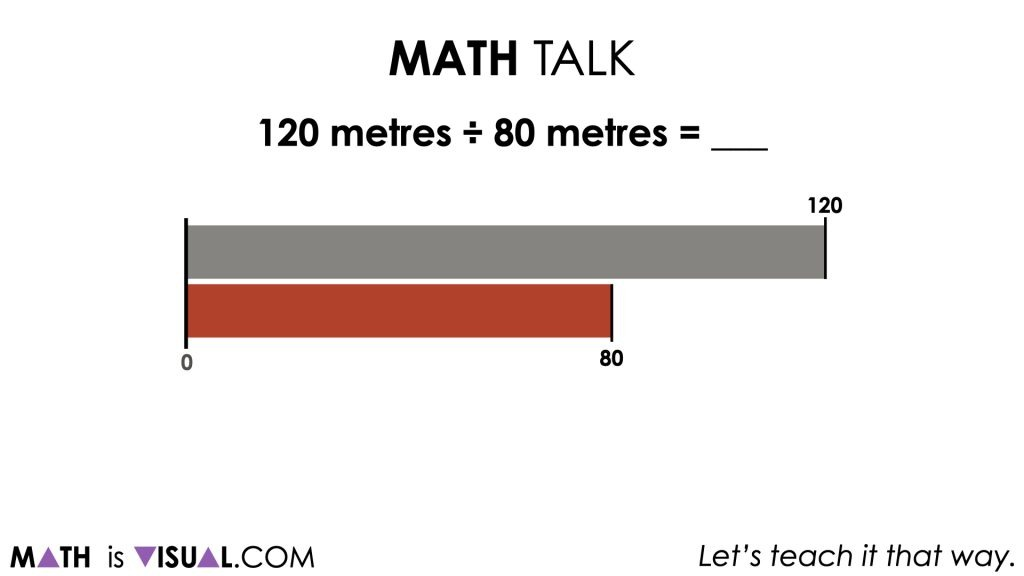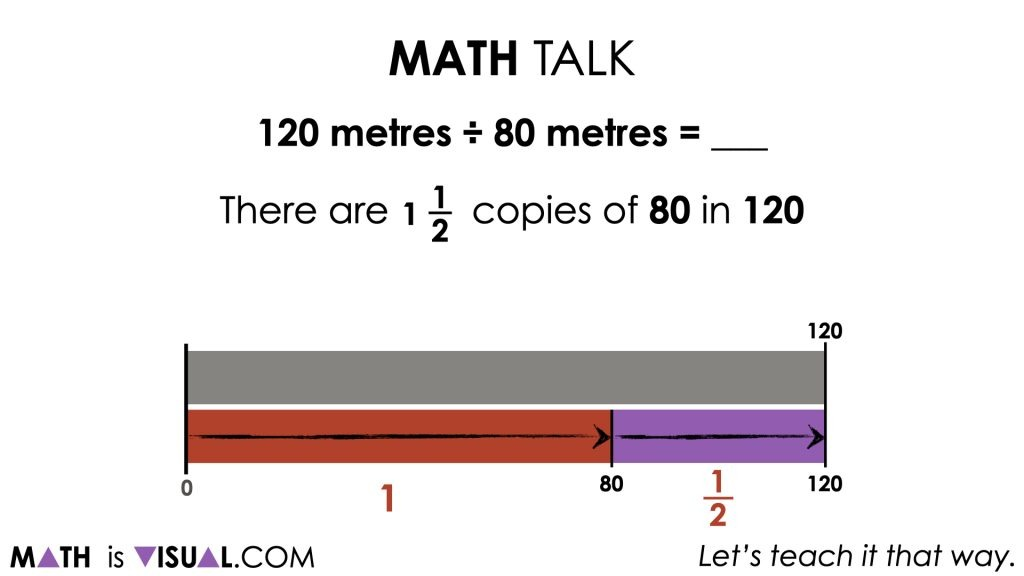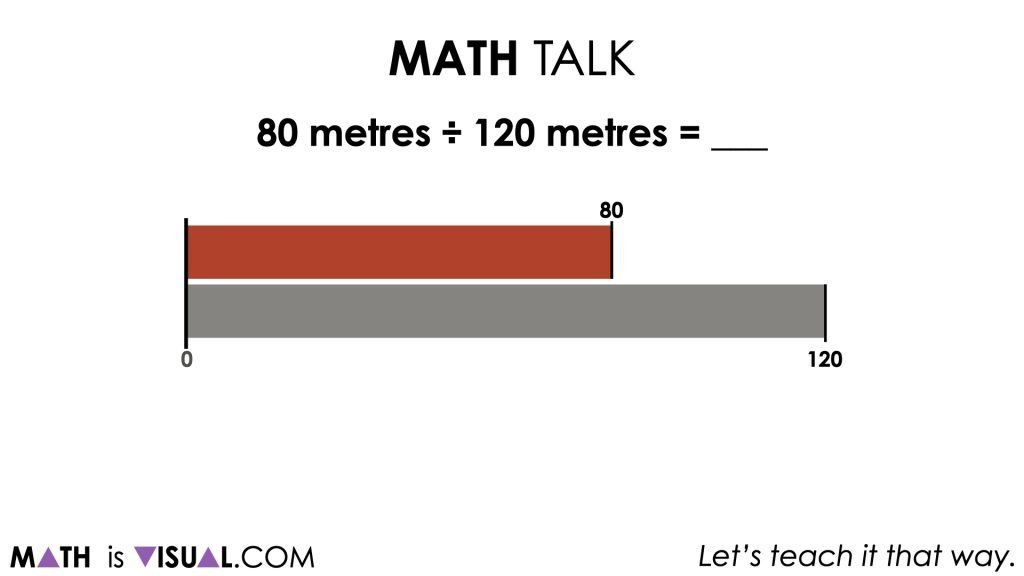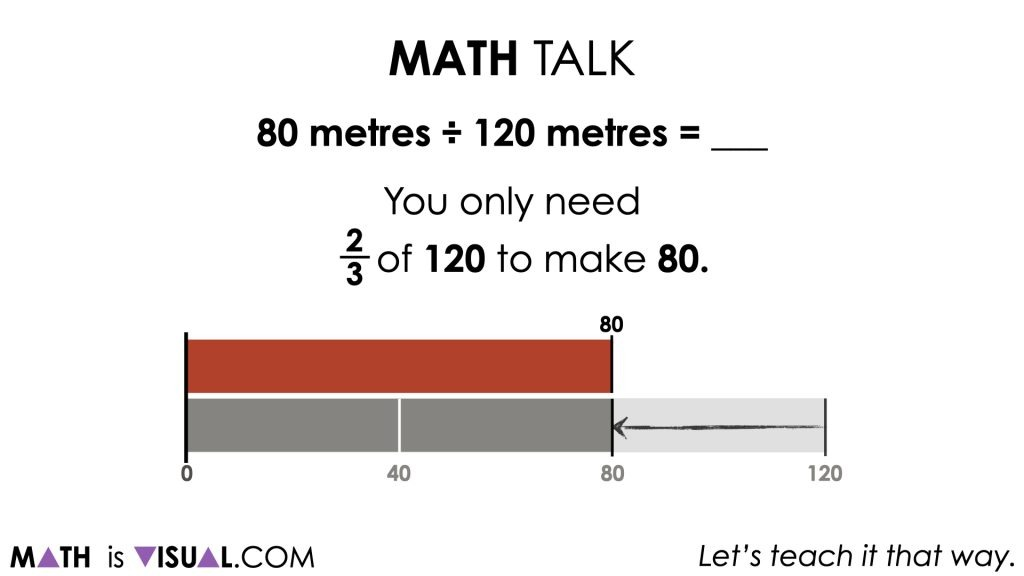# Scale Factors of Multiplicative Comparison Ratios

Build flexibility with visual math talk prompts involving revealing the scale factors of multiplicative comparison ratios using stacked bar models.

## In This Set of Math Visual Prompts…

Students will explore quotative division sentences involving quantities of the same unit to reveal a scale factor.

## Intentionality…

This set of visual math talk prompts is taken from the Math Talk section of Day 4 in the Make Math Moments Problem Based Unit called Rice Ratios. The purpose of the Math Talk is to reinforce key concepts and big ideas from this problem based math unit including:

• There are two types of ratios; composed unit and multiplicative comparison;
• A composed unit is often (not always) a ratio with two distinct units;
• A composed unit can be scaled in tandem;
• Equivalent ratios are derived from the same rate;
• When you divide a composed unit through partitive division, you reveal a rate.
• Quotative division is applied when trying to determine a scale factor between two quantities/measures of the same unit (i.e. rice and rice, water and water);
• When you invert the dividend and the divisor in a quotative division sentence, the quotient (the revealed scale) has a reciprocal relationship (i.e., 4 1 = 4 ,1 4 = ¼ ).

Unsure about some of the terminology listed above?

## Preparing to Facilitate

In today’s visual math talk, we will be presenting the following quotative division sentences one at a time. Since we are dividing quantities of the same unit, the type of division is quotative and the resulting quotient will represent a scale factor. Encourage the use of an area, volume or linear model to justify each equation.

120 metres ÷ 80 metres

80 metres ÷ 120 metres

An additional four (4) prompts are shared in the Teacher Guide from Day 4 of the Rice Ratios problem based math unit

You might consider modelling each of these division sentences using a stacked bar model as shown in the visual math talk prompt silent solution animations shared below.

## Problem #1:

The first problem in the visual math talk prompt asks students to divide 120 metres by 80 metres. Since we are dividing quantities with the same units (metres by metres), we know that this is quotative division since we are asking:

How many units of 80 metres are in 120 metres?

Leveraging a linear model to scale for this quotative division problem will allow students to see that there is at least one full unit of 80 metres in 120 metres, but less than two full units.Making a second copy of 80 metres and placing it in the gap between 80 metres and 120 metres will reveal that adding an additional half 80 metres will “fill the gap”.Therefore, there are 1 and 1 half or 3 half copies of 80 metres in 120 metres.

## Problem #2:

Now, we will switch the dividend and divisor quantities by showing this next visual math talk prompt.

Students may choose to leverage their first model to visualize how many copies of 120 metres are in 80 metres. They will quickly realize that it is less than 1 copy.

If students respond with an answer of zero, push students to be more precise. Asking them questions like “is it exactly zero or could a fractional amount work?” might be helpful.Just by looking at their model – assuming it is reasonably to scale – students should note that there is more than half of 120 metres in 80.

Some students might benefit from using paper strips to represent their bar model and using folds to partition until they land on partitioning 120 metres into thirds.Therefore, there is 2 thirds of 120 metres in 80 metres.

## Want to Explore These Concepts & Skills Further?

Four (4) additional math talk prompts are available in Day 4 of the Rice Ratios problem based math unit that you can dive into now.

Why not start from the beginning of this contextual 5-day unit of ratios and rates real world lessons from the Make Math Moments Problem Based Units page.

Did you use this in your classroom or at home? How’d it go? Post in the comments!

Math IS Visual. Let’s teach it that way.